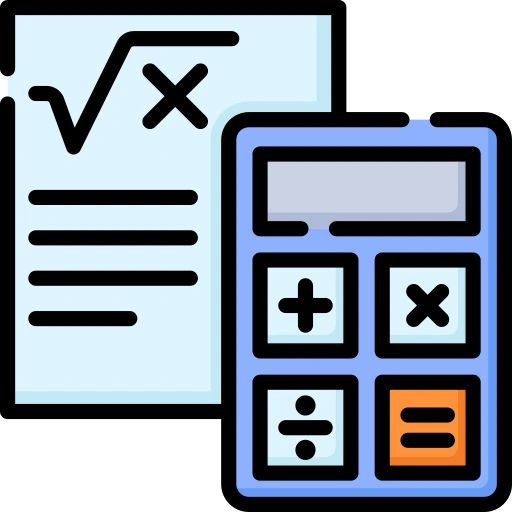# Tips And Tricks And Shortcut For Logarithm

## Tips Tricks and Shortcuts Of Logarithms

Logarithm is the way to represent exponential expression as shown in the example .

Example : a x = y is a exponential expression And it can also be written as x = log a y . which is a logarithm expression.### Tips and Tricks and Shortcuts to solve Logarithm

• The first question which arises in our mind is What is Logarithm?
• Definition : The Logarithm is the inverse function of exponentiation.
• logarithm is a power to which a number must be raised to get another number.
• Logarithm was introduced by John Napier in the early 17th century to simplify calculations.
• A logarithm is of two types:-
• Common Logarithm
• Natural Logarithm
• Common Logarithm- Logarithm with base 10 is Common logarithm.
• Natural Logarithm-  Logarithm with base e is Natural Logarithm.

### Example of Logarithm :

Question: log 100 = 2
Taking LHS

log 102

= 2 log 10

= 2 × 1     (∴ Log 10 = 1 )

= 2 .

• Let’s have a look on some question that will help better in understanding Logarithm.

### Related Banners

Get PrepInsta Prime & get Access to all 200+ courses offered by PrepInsta in One Subscription

Question 1 Which of the following statement is not correct?

Option

A) log (1 + 2 + 3) = log 1 + log 2 + log 3

B) log( 2+3) = log (2×3)

C) log10 1 = 0

D) log10 10 = 1

Solution:

a)  log( 1+2+3) = log6 = log (1×2×3) = log1+ log2+log3

b) log (2 + 3) = log 5 and log (2 x 3) = log 6 = log 2 + log 3

log (2 + 3) ≠ log (2 x 3)

c) Since,  loga 1=0,
so log10 1=0

d) Since, loga a = 1
so, log10 10 = 1.

Question 2 If X is an integer then solve(log2 X)2– log2x4-32 = 0

Solution :    Let us consider,(log2 X)2– log2x4-32 = 0 —— equation 1

let log2 x= y

equation 1 = y2– 4y-32=0

y2-8y+ 4y – 32= 0

y(y-8) + 4(y-8) =0

(y-8) (y+4)= 0

y=8, y= -4

log2X=8 or, log2X = -4

X= 28 = 256, 0r 2-4 = $\frac{1}{16} \$

Since, X is an integer so X = 256.

Question 3 Solve for x: $\log_{2}(x + 3) + log_{2}(x – 1) = 3$

Solution: Combine the logarithms using the logarithm property log(a) + log(b) = log(a * b):
$\log_{2}((x+3)\times(x-1)) = 3$

$2^3=(x+3)\times(x-1)$

$8=(x+3)\times(x-1)$

$8=x^2+2x-3$

$x^2+2x-11=0$

(x+4)(x-2)=0

x = -4,2

## Get over 200+ course One Subscription

Courses like AI/ML, Cloud Computing, Ethical Hacking, C, C++, Java, Python, DSA (All Languages), Competitive Coding (All Languages), TCS, Infosys, Wipro, Amazon, DBMS, SQL and others